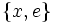# Permutably complemented is not transitive

This article gives the statement, and possibly proof, of a subgroup property (i.e., permutably complemented subgroup) not satisfying a subgroup metaproperty (i.e., transitive subgroup property).
View all subgroup metaproperty dissatisfactions | View all subgroup metaproperty satisfactions|Get help on looking up metaproperty (dis)satisfactions for subgroup properties
Get more facts about permutably complemented subgroup|Get more facts about transitive subgroup property|

## Statement

### Statement with symbols

It can happen that we have groups$H \le K \le G$ such that$H$ is a permutably complemented subgroup of$K$ and$K$ is a permutably complemented subgroup of$G$, but$H$ is not a permutably complemented subgroup of$G$.

## Proof

### Example of the dihedral group

Further information: dihedral group:D8

Let$G$ be the dihedral group of order eight; specifically:$G = \langle a,x \mid a^4 = x^2 = e, xax^{-1} = a^{-1} \rangle$.

Let$H$ be the center of$G$:$H = \{ a^2 , e \}$.

Let$K$ be the elementary Abelian subgroup generated by$a^2$ and$x$, so$K = \{ e, a^2, a^2x, x \}$.

We have:

•$H$ is permutably complemented in$K$: The subgroup$\{ x, e\}$ is a permutable complement to$H$ in$K$.
•$K$ is permutably complemented in$G$: The subgroup$\{ ax , e\}$ is a permutable complement to$K$ in$G$.
•$H$ is not permutably complemented in$G$: This can be seen by inspection, but it also follows from a more general fact about nilpotent groups: every nontrivial normal subgroup of a nilpotent group intersects the center nontrivially. A permutable complement to the center must be a nontrivial normal subgroup, and hence such a thing cannot exist in a nilpotent group.